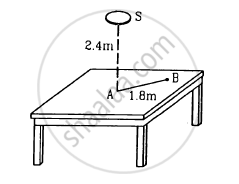Department of Pre-University Education, KarnatakaPUC Karnataka Science Class 12

# Figure Shows a Small Diffused Plane Source S Placed Over a Horizontal Table-top at a Distance of 2.4 M with Its Plane Parallel to the Table-top. - Physics

Sum

Figure shows a small diffused plane source S placed over a horizontal table-top at a distance of 2.4 m with its plane parallel to the table-top. The illuminance at the point Adirectly below the source is 25 lux. Find the illuminance at a point B of the table at a distance of 1.8 m from A.#### Solution

Let x be the distance moved.

Let the illuminance of the candle and the lamp at a distance 1 m be IC and ILrespectively. According to question,

E=I_L/(0.8)^2=I_c/(0.2)^2

rArr I_L/I_c=16

Again after the wrapping the paper around

49/100I_L/(0.8+x)^2=I_c/(0.2)^2

Either,

x = -0.24

Or,

x = -1.36 this value is not acceptable because it is more than 0.8

so, x = 0.24m (-ve sign denotes distance is to be decreased)

x = 24cm

Concept: Light Process and Photometry
Is there an error in this question or solution?

#### APPEARS IN

HC Verma Class 11, Class 12 Concepts of Physics Vol. 1
Chapter 22 Photometry
Exercise | Q 14 | Page 455
Share